# Bifurcations with dihedral symmetry

Any function invariant under the Dn action on the plane is a function of the two fundamental homogeneous polynomials:
$$N=x^2+y^2 = |z|^2,\quad \mbox{and} \quad P = \mathrm{Re}(z^n)$$ The following diagrams show level sets of the functions $$h_\lambda(z) = \lambda N +aN^2 + bP.$$ So $$h_\lambda$$ has a local min at the origin when $$\lambda\gt0$$.

We need to assume $$b\neq0$$, otherwise the function $$h_0$$ does not have an isolated singularity.
And w.l.o.g, we can assume $$b>0$$ (after a possible rotation in the plane).
(If $$b=0$$ we would need to take into account higher order terms such as $$P^2$$).
Mostly we take $$a=b=1$$, but for $$n=4$$ we must take $$a\ne\pm b$$.

Dn λ<0 λ>0 discussion
n=3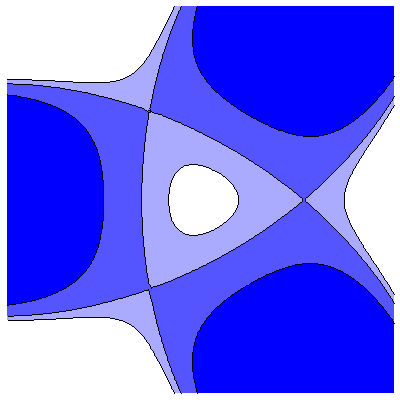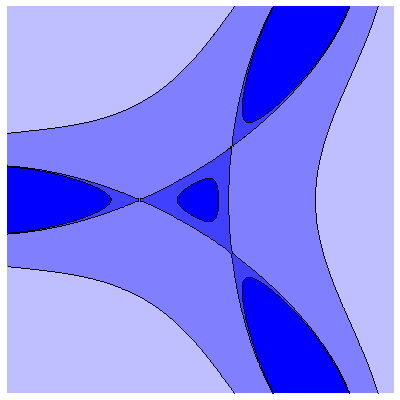On both sides of the bifurcation, there are 3 saddle points; this is a traditional transcritical bifurcation
n=4 (i)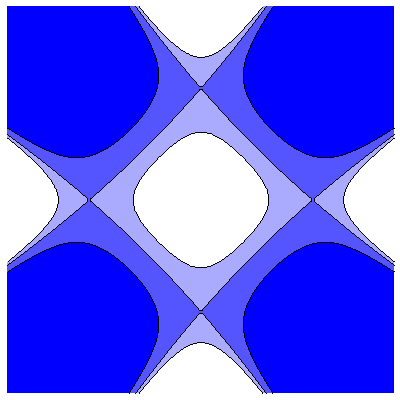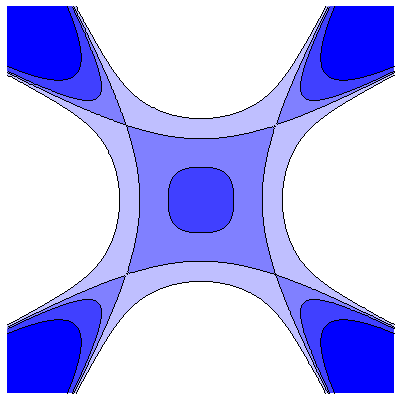(a<b) On both sides of the bifurcation, there are 4 saddle points; this is a traditional transcritical bifurcation
n=4 (ii)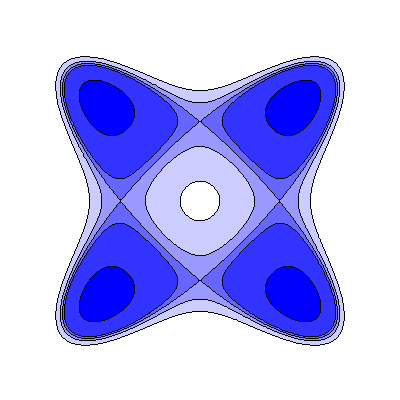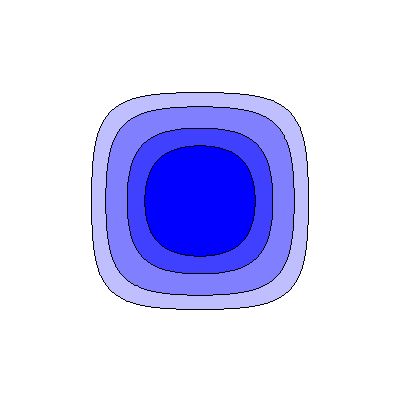(a>b) On one side there are 4 saddles and 4 local minima, and on the other no bifurcating critical points
n=5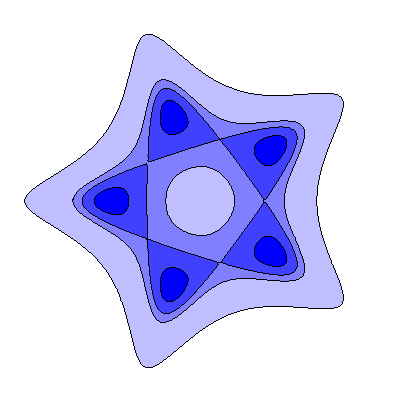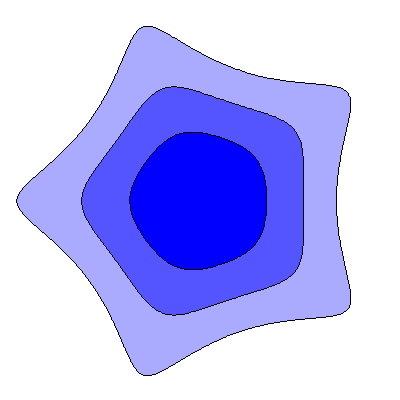On one side there are 5 saddles and 5 local minima, and on the other no bifurcating critical points
n=6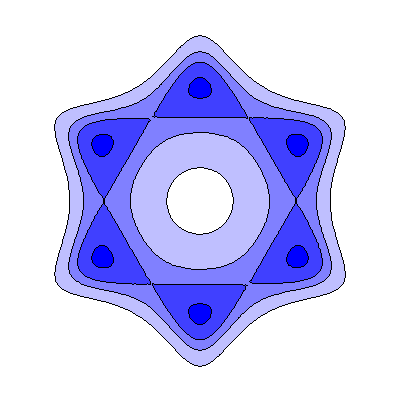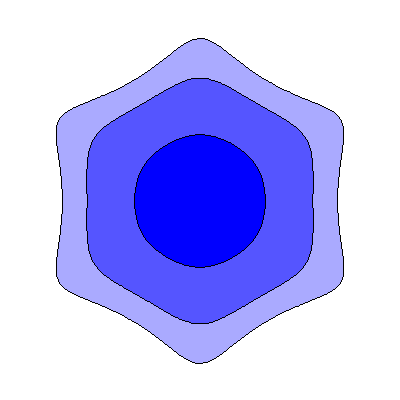On one side there are 6 saddles and 6 local minima, and on the other no bifurcating critical points
In the diagrams, if points $$p$$ and $$q$$ are such that $$p$$ is coloured bluer than $$q$$, then $$h(p) \lt h(q)$$.

Remark: Notice how $$n = 3$$ and $$n = 4(i)$$ are similar, while $$n = 4(ii)$$ and $$n \gt 4$$ are similar.
The general pattern depends on which of the terms $$aN^2$$ or $$bP$$ dominates.
Notice that all the bifurcating critical points lie in lines of symmetry;
this means that the solutions they represent in a given problem will have an order 2 symmetry.

James Montaldi